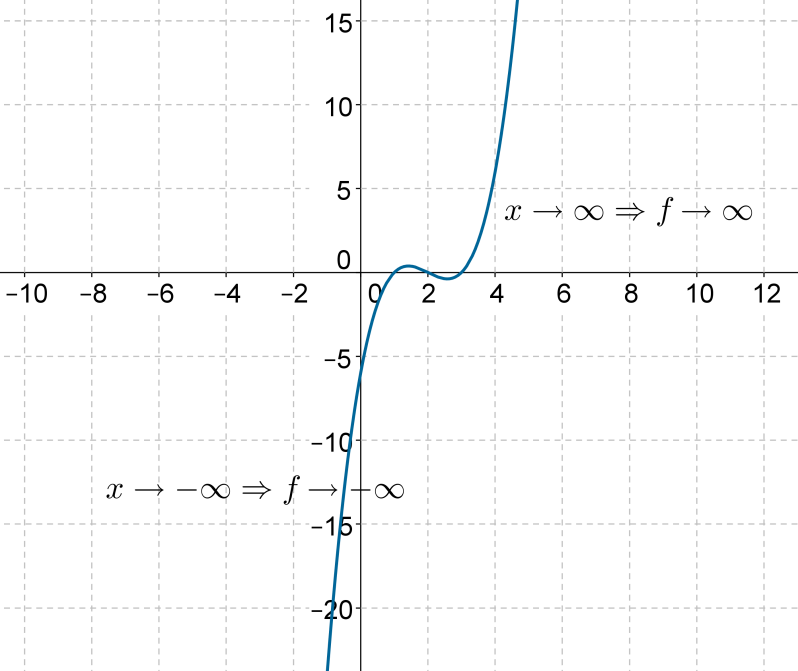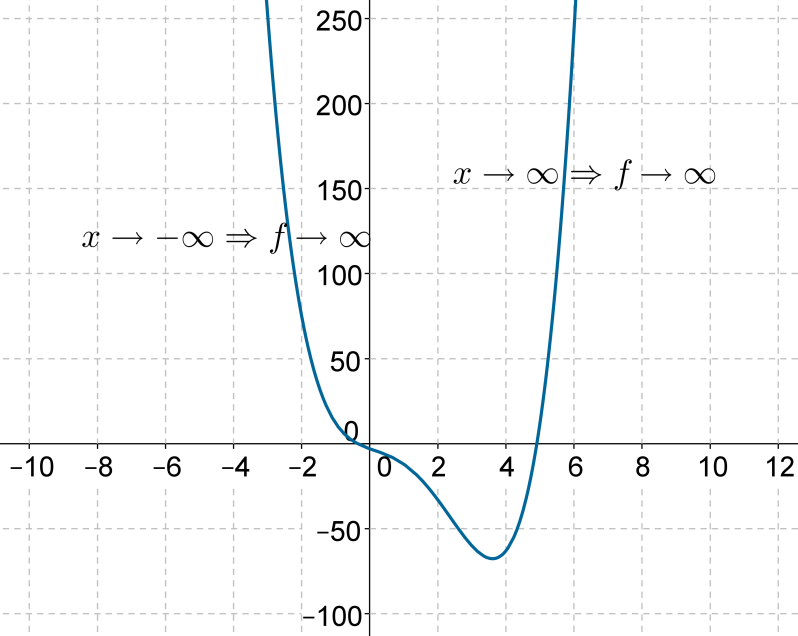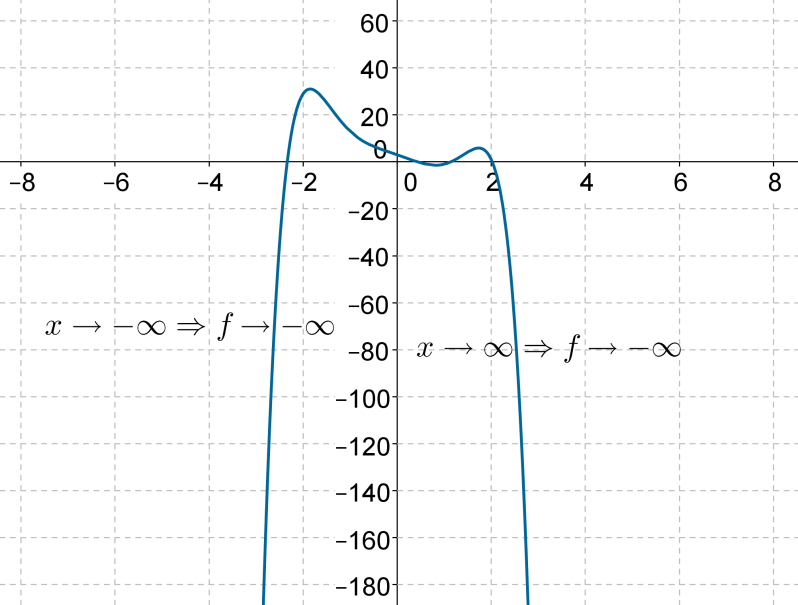# Polynomial Functions

Go back to  'Functions'

We have already discussed polynomials in detail. A polynomial function of degree n has the following definition:

$f\left( x \right) = {a_0}{x^n} + {a_1}{x^{n - 1}} +{a_2}{x^{n - 2}} + ... + {a_{n - 1}}x + {a_n}$

The coefficient of the highest degree term should be non-zero, otherwise f will be a polynomial of a lower degree.

All polynomial functions are defined over the set of all real numbers. In other words, the domain of any polynomial function is  $$\mathbb{R}$$.

The range of a polynomial function depends on the degree of the polynomial. Let us see how. Consider the polynomial

$f\left( x \right) = {a_0}{x^n} + {a_1}{x^{n - 1}} +{a_2}{x^{n - 2}} + ... + {a_{n - 1}}x + {a_n}$

once again. When x takes on very large values, the highest degree term $${a_0}{x^n}$$dominates in magnitude compared to the other terms. For example, let

$f\left( x \right) = {x^5} + {x^4} + 1$

Take a really large value for x,say $$x = {10^6}$$. For this value of x,the highest degree term has a magnitude of $${10^{30}}$$,while the second term has a magnitude of (only) $${10^{24}}$$. The first term is larger than the second term by a factor of $${10^6}$$ or 1 million. Clearly, for really large values of x, only the highest degree term matters – the other terms have relatively negligible magnitudes.

Now, suppose that the degree of the $$f\left( x \right)$$ polynomial is odd. Also,suppose that the value of $${a_0}$$ in the highest degree term $${a_0}{x^n}$$ is positive.

We make the following observations:

1. When x takes on large positive values, then $${a_0}{x^n}$$also takes on large positive values. We can make $${a_0}{x^n}$$ as large as possible by taking a sufficiently large value of x. Thus, we can say that as x tends to infinity, the term $${a_0}{x^n}$$ also tends to infinity: as $$x \to \infty$$, $${a_0}{x^n} \to \infty$$.

2. When x takes on large negative values, $${a_0}{x^n}$$also takes on large negative values, because the power of x in this term(which is n) is odd. That is: as $$x \to - \infty$$,   $${a_0}{x^n} \to - \infty$$.

Since the value of f for large values of x is mostly influenced by the highest degree term, we can say that:

1. As $$x \to \infty$$,  $$f \to \infty$$.

2. As $$x \to - \infty$$,  $$f \to - \infty$$.

Also, any polynomial function has a continuous variation, as mentioned earlier (there are no breaks in the graph).This means that the curve for f will vary (continuously) from a y-value of negative infinity to a y-value of positive infinity. In other words,the range of f is $$\mathbb{R}$$.

If the coefficient $${a_0}$$ in the highest degree term $${a_0}{x^n}$$ is negative, then.

1. As $$x \to \infty$$,  $$f \to - \infty$$.

2. As $$x \to - \infty$$,  $$f \to \infty$$.

Once again, f will have a range of   $$\mathbb{R}$$.

We conclude that for any polynomial function with an odd degree, the range will be  $$\mathbb{R}$$ . Let us see some examples.

The following figure shows the plot of.

$f\left( x \right) = {x^3} - 6{x^2} + 11x - 6$Note that the scale of the two axes is different.

The following figure shows the plot of the fifth degree polynomial

$f\left( x \right) = - 3{x^5} + 7{x^3} - 10x + 4$In both cases, it is easy to see that the range of the polynomial function is  $$\mathbb{R}$$.

What can we say about the range of a polynomial function of even degree? We have already seen that the curve for a quadratic function is a parabola opening upward or downward, depending upon whether the coefficient of the square term is positive or negative(respectively). This means that the range of a quadratic function is not $$\mathbb{R}$$  but a proper subset of $$\mathbb{R}$$.

The following figure shows the plot of the fourth degree polynomial function

$f\left( x \right) = {x^4} - 5{x^3} + 2{x^2} - 7x -3$Both the arms of the curve tend towards infinity. On the negative side, there is a certain value below which the curve does not go. The range of this function is therefore a subset of  $$\mathbb{R}$$ - from the minimum value to positive infinity.

Why do polynomial functions with even degrees don’t have ranges of  $$\mathbb{R}$$? If the coefficient $${a_0}$$ in the highest degree term $${a_0}{x^n}$$ is positive,then f will tend to positive infinity on both sides. That is.

1. As $$x \to \infty$$,    $$f \to \infty$$.

2. As $$x \to - \infty$$,    $$f \to \infty$$.

Thus, the curve never goes towards negative infinity. It has a finite minimum value.

On the other hand, if the coefficient $${a_0}$$ in the highest degree term  $${a_0}{x^n}$$ is negative, then f will tend to negative infinity on both sides.

1. As$$x \to \infty$$,     $$f \to - \infty$$.

2. As$$x \to - \infty$$,    $$f \to - \infty$$.

Thus, f will have a finite positive value. The following figure shows the plot of the sixth-degree polynomial function

$f\left( x \right) = - {x^6} + 2{x^4} - {x^2} - 3$Clearly, f has a finite maximum value (somewhere between 20 and 40). The range of f will be negative infinity to this maximum value.

Let us now summarize our discussion on polynomial functions.

1. The graph of any polynomial function has a continuous curve.

2. The domain of any polynomial function is $$\mathbb{R}$$.

3. The range of a polynomial function depends upon its degree:

1. If the degree is odd, then the range is  $$\mathbb{R}$$.

2. If the degree is even, then

1. If the coefficient of the highest degree term is positive, the range will be a certain minimum value to positive infinity.

2. If the coefficient of the highest degree term is negative, the range will be negative infinity to a certain maximum value.

4. A polynomial function will assume every value in its range, as the curve for the function is continuous.

Example 1: What can you say about the range of a 101-degree polynomial? Will it be  $$\mathbb{R}$$ , or a subset of $$\mathbb{R}$$ ?.

Solution: Since the degree of the polynomial is odd, its range will be $$\mathbb{R}$$ .

Functions
Functions
grade 10 | Questions Set 2
Functions
Functions
grade 10 | Questions Set 1